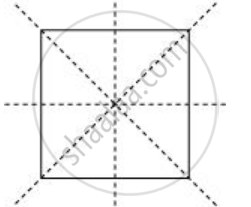Share

# State the Number of Lines of Symmetry for a Square Figure - CBSE Class 7 - Mathematics

ConceptLines of Symmetry for Regular Polygons

#### Question

State the number of lines of symmetry for A square figure

#### Solution

There are 4 lines of symmetry in a square.Is there an error in this question or solution?

#### APPEARS IN

NCERT Solution for Mathematics for Class 7 (2018 to Current)
Chapter 14: Symmetry
Ex. 14.10 | Q: 7.04 | Page no. 270
Solution State the Number of Lines of Symmetry for a Square Figure Concept: Lines of Symmetry for Regular Polygons.
S